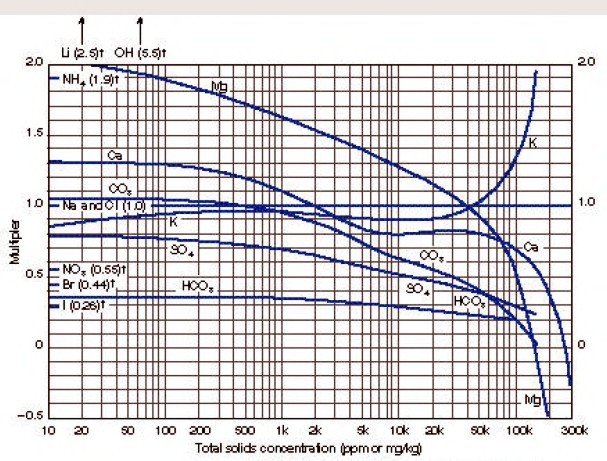WATER SALINITY and Total dissolved solids
The salinity of a water sample can be described numerically in several different ways, each with a confusing set of measurement units. This page covers the summation of water chemistry analysis data and conversions between total dissolved solids (TDS), equivalent NaCl content, and chloride content. Conversion of salinity to water resistivity is covered on the next Section in this Chapter.Equivalent NaCl Water Salinity from Water Analysis
The resistivity of a water sample can be calculated from its chemical analysis. To do this, an equivalent NaCl concentration must be determined based on the ionic activity of each ion.Enter chart with total solids concentration of the sample in ppm (mg/kg) to find weighting factors for each ion present. The concentration of each ion is multiplied by its weighting factor, and the products for all ions are summed to obtain equivalent NaCl concentration.

The math is pretty simple:
1: TDS = SUM (IONi)
2: WSe = SUM (IONi * FACTRi)

Where:
TDS = total dissolved solids (ppm)
IONi = ion concentration of ith component (ppm)
FACTRi = multiplier factor for ith component (ppm)
WSe = equivalent NaCl concentration (ppm)NUMERICAL EXAMPLE:
Assume formation-water sample analysis
460 ppm Ca,
1400 ppm SO4
19,000 ppm Na plus Cl.

Total dissolved solids concentration
TDS = 460 + 1400 + 19,000 = 20,860 ppm
Entering the chart with this total solids concentration
Ca multiplier = 0.81
SO4 multiplier = 0.45
Na+CL multiplier = 1.00
Equivalent NaCl concentration = 460 ´ 0.81 + 1400 ´ 0.45 + 19,000 ´ 1.0 = 20,000 ppm.Water Salinity from Chloride Content
Sometimes salinity is reported at the well site in ppm Chlorides instead of ppm NaCl equivalent.
6: WSa = Ccl * 1.645

Where:
Ccl = water salinity (ppm Cl)
WSa = water salinity (ppm NaCl)COMMENTS:
Use this relationship when chloride content of the water sample is known. Usually Cl content is derived at the well site from a drill stem test recovery. It is useful as a first approximation until the water sample is analyzed more accurately at a laboratory. The relationship is for pure NaCl solutions and the factor may be higher or lower if other ions are present.NUMERICAL EXAMPLE:
1. Chloride concentration to salinity.
WS = 11,600 ppm Cl * 1.645 = 19,000 ppm NaCl

Page Views ---- Since 01 Jan 2015